# Design and Analysis of Sen Transformer Using FEM and No Load Loss Calculation

Design and Analysis of Sen Transformer Using FEM and No Load Loss Calculation
Saradar Vallabhbhai National Institute of Technology, Surat 395007, India

Corresponding Author Email:
d17el002@eed.svnit.ac.in
Page:
493-500
|
DOI:
https://doi.org/10.18280/mmep.070321
17 January 2019
|
Accepted:
14 July 2020
|
Published:
30 September 2020
| Citation

OPEN ACCESS

Abstract:

The “Sen Transformer (ST)” was introduced by Dr. Kalyan Sen for controlling the power flow in transmission line. The ST provides true need of optimal power flow control that is characterized as a reliable, efficient and least expensive device. The primary of ST is connected in shunt with the transmission line, which is energized transformer core continuously. As ST is connected with the transmission line for 24 hours, a constant no load loss of energy occurs in ST. Due to an expressive loss of energy and an unfortunate influence of losses on the performance of ST, significant core loss (no load loss) is examined as a judgmental factor. Not only with pure input supply, but also with harmonics in supply and even in over excited condition, the behavior of ST should be examined. Finite Element Method (FEM) has been used over here to simulate core loss of ST and investigate behavior of flux density, magnetic field, core losses etc. with sinusoidal as well as non- sinusoidal input sources. Two different structures of ST are considered, which is used for distribution line, in proposed work and the effects of non sinusoidal voltage on no load loss have been investigated and compared for both structures using Ansys Maxwell.

Keywords:

Sen Transformer, no load loss, FEM, flux density, power flow controller, magnetic equivalent circuit

1. Introduction

The “Sen Transformer (ST)” is a combination of a conventional transformer and a tap changer unit. The ST has two modules, 1) Primary winding 2) Series winding. The primary winding is shunt connected unit, often referred as excitation winding. The Series connected is referred as compensation winding . The ST can control both active and reactive power like UPFC. It is well known from the operation of UPFC that to control the active and reactive power flow in the line, value and phase angle of injected voltage should be controlled. In UPFC this is done by controlling the switching of power devices. In ST to do this different combination of secondary windings are used. There are total nine windings equally distributed on each phase. By properly selecting the winding combination voltage can be injected at any angle between 00 to 3600 with a particular value of voltage. The ST has numerous advantages in the power system like power flow control, voltage regulation, loss minimization etc. and hence it is indispensable for power system security and asset management to do a detailed study of Sen Transformer. A detailed study of ST starts with modeling and FEM analysis. For modeling of ST, hysteresis and eddy current behavior, accuracy of core saturation, residual fluxes, inter-phase coupling all are significant parameters . Geometry of ST is essential to develop a model and analyse mentioned phenomenon. Behavior of localized fluxes in ST core is necessary to understand in detail for developing petite cores with higher efficiency. If sufficient information is known for inter-relationship of the magnetic characteristics of materials for core such as flux distribution in a core, hysteresis, eddy current effects and even not only with different core material but also with a petite core with greater efficiency, than better ST would be established as a transformer . It is possible to manipulate hand on solutions of Maxwell’s equations in 2D or 3D design of ST by Finite Element Method [FEM]. The FEM simulation software permits for designing of capricious geometry with the constraint that acquire axial symmetry which produces such a characteristic as resistive losses in insulation and in windings due to non linearity of a magnetic system core or eddy current . Design of ST is done considering by considering the worst situation. From the experience of transformer design, even though all measurements are properly calculated, there is a possibility of defect in transformer. Some problems are created due to mechanical stress because of power conditions, when transformer is attached with the electrical network . In transformer windings, these mechanical stresses are produced by transient electromagnetic forces in radial and axial directions. A variety of undesirable effect in the system may occur due to the high magnitude current (inrush current, transient current due to disturbances in electrical power network) which produces electromagnetic transient . Hence, the study of the transients in ST is essential. The transformer’s electromagnetic behavior is shown by the non-linear Maxwell equations. Additional losses due to the power quality problem can be limited as a real and reactive power of the transformer is preferable, before any consequential damage because of supplementary temperature rises . The FEM gives its solution and sanction additional precise computation of some transformer quantities, or else susceptible to error when experimental as well as analytical relation are used. Yu developed novel software for analyzing flux and iron losses to optimize design of transformer. The finite element technique has been developed for computation of two dimensional magnetic field and loss distribution. The optimized design of three phase, three limb transformer cores has been done . Stadler  discussed the influence of winding layout on the core- losses and the leakage inductance in high frequency transformers. For the magnetization (clear this sentence), a method of summation is used to solve integral equations and some design guidelines are illustrated to optimize various components.

The presence of harmonic current component can cause additional losses in the windings and other structure parts has been claimed by Lin  (Is it necessary to indicate these years). Hernandez developed a hybrid system to design distribution transformer, in which Knowledge Based System (KBS) 2D FEM is used to figure out electromagnetic field in transformer. For the analysis, FEM is used as a numerical technique that solve partial differential equations and KBS is used as a software product that implies (or applies) established artificial intelligent techniques. To investigate magnetizing current and iron loss, a 2D Cartesian model is used to compute eddy current losses and leakage reactance (are these two separate sentences). An axisymmetric model is used in simulation of 1500 KVA,13200-200 V, delta-star, oil field distribution transformer . Yazdani-Asrami investigated no load loss calculation of distribution transformer by using 3-D finite element analysis in the case of sinusoidal and non-sinusoidal voltage supply. It is well known that in distributing transformer No-load loss dominates the total energy loss due to its 24 hour operation in power system. Because of undesirable impact of losses and high significance of energy, the no load loss is considered critical factor for the aging of transformer. The FEM is used to simulate impact of non sinusoidal voltage effect on transformer by analyzing various parameters like flux density, flux intensity, no load loss under various input conditions .

The finite element method is based on solution of Maxwell equations. The transformer electromagnetic field solution is very difficult to obtain. Analytical methods are also not useful due to magnetic non linearity. To design a Sen Transformer, it is necessary to estimate the reactance, the flux distribution in iron core, the leakage fields and core loss. Therefore, practical realization of the transformer is complex even though it seems like simple device. Turns of transformer winding are made up of small rectangular copper conductor strands, which are linked by alternating magnetic fields and its losses are estimated through a magnetostatic solution. The FEM is adopted to determine the losses in each turn of ST windings to examine hot spot temperature.

The FEM can perform significant investigation of flux distribution in ST core, as it becomes simple through the numerical field calculations process. Therefore, the inter relation between the magnetic characteristics of core materials of those of ST core can be scrutinized analytically. In FEM, the effect of only one factor is to be examined but in experimental results of interrelation uses more money, labor and time. In the presented work, two different structure of STs are modeled and core losses are examined for varying inputs.

2. FEM and Maxwell’s Equations

The ordinary as well as partial differential equations can be solved by mathematical method i.e. finite element method. The FEM has the potential to simplify complex problems which are expressed in differential equation form, as it is a numerical method. The FEM is having infinite practical applications for designing problems, as the differential equations form naturally in virtually all fields of the physical sciences.

The foundation of FEM is a mathematical theorem having a solid theoretical foundation. It is based on Maxwell’s equations that assure an asymptotic inflation of the accuracy of the field calculation towards the exact solution. The solution process decreases as the size of the finite elements used increases. For time domain solutions the spatial discretization of the problem must be refined in a manner such that it coordinates with the time steps of the calculation according to estimated time constants of the solution (such as magnetic diffusion time constant). By solving Maxwell's equations, Maxwell solves the electromagnetic field problems in a finite region of space with significant boundary conditions. In order to obtain a solution with assured existence it is taking necessary user specified initial conditions. The geometry of the problem is discretized automatically into tetrahedral elements, to obtain the set of algebraic equations to be solved. All the solid geometry of model is meshed automatically by the masher, which form tetrahedral elements. The assembly of all tetrahedral elements are referred to as the finite element mesh of the model or simply the mesh. The unknown characteristic for the field being calculated, which are represented as polynomials of second order inside each tetrahedral. Therefore, in regions with rapid spatial field variation, the mesh density needs to be increased for good solution accuracy.

To perform specific engineering task, as well as electrical system design based on different phenomena such as electrical, electromechanical or electrochemical, the Maxwell's equations perform a vital role.

The transformer induces an electromagnetic field, when it is excited by an ac voltage source. An electromagnetic field is mentioned by Maxwell’s equations by relating five vector fields, mentioned as,

(i) Magnetic flux density B (T)

(ii) Magnetic field H (A/m)

(iii) Electrical field E (V/m)

(iv) Electrical displacement (D)

(v) Electrical current density J (A/m2)

Maxwell’s equations are,

$\nabla \times H-\sigma v \times B=J+\partial D / \partial t$      (1)

$\nabla \times E=-\partial B / \partial t$     (2)

$\nabla \cdot B=0$     (3)

$\nabla \cdot D=\rho$     (4)

where, ρ=electric charge density

v=velocity of the medium

σ=electric conductivity

The Maxwell’s equations have to expand with essential material equations, which are,

$\mathrm{B}=\mu H$     (5)

$D=\in E$      (6)

$\mathrm{J}=\sigma E$       (7)

where, μ=magnetic permeability

$\in=$electric permeability

σ=electric conductivity

Magnetic and dielectric relationship is show by Eqns. (5) & (6) and Eq. (7) shows the ohm’s law.

3. Core Loss and B-H Curve

For a transient solution type, core loss i.e. no load loss includes hysteresis losses and eddy current losses. The core loss is carried out by post-processing calculation, which depends on previously calculated transient magnetic field quantities.

3.1 Core loss coefficients for transformer core

In the frequency domain, the core loss is determined with sinusoidal flux conditions as following,

$P_{v}=P_{h}+P_{c}+P_{e}$     (8)

$P_{v}=k_{h} f\left(B_{m}\right)^{2}+k_{c}\left(f B_{m}\right)^{2}+k_{e}\left(f B_{m}\right)^{1.5}$     (9)

The core loss is further transforms to the following equation with DC component present in the flux density:

$P_{v}=C_{d c} k_{h} f\left(B_{m}\right)^{2}+k_{c}\left(f B_{m}\right)^{2}+k_{e}\left(f B_{m}\right)^{1.5}$     (10)

where, Bm = maximum amplitude of the AC flux component,

f = Frequency,

Kh = Hysteresis core loss coefficient,

Kc= Eddy-current core loss coefficient,

Ke = excess core loss coefficient.

Here,

$C_{d c}=\sqrt{K_{d c}\left|B_{d c}\right| / B_{m}+1}.$

where, Bdc = DC flux component,

Kdc = Coefficient considering DC flux bias effects.

3.2 Core loss coefficient extraction

To determine Kh, Kc, and Ke a computation algorithm is implemented and the equations are as follows.

$P_{v}=P_{h}+P_{c}+P_{e}$     (12)

$P_{v}=k_{h} f\left(B_{m}\right)^{2}+k_{c}\left(f B_{m}\right)^{2}+k_{e}\left(f B_{m}\right)^{1.5}$     (13)

$P_{v}=K_{1} B_{m}^{2}+K_{2} B_{m}^{1.5}$      (14)

where,

$K_{1}=K_{h} f+K_{c} f^{2}$     (15)

$K_{2}=K_{e} f^{1.5}$      (16)

The loss coefficient of eddy-current is calculated directly as following,

$K_{c}=\pi^{2} \sigma \frac{d^{2}}{6}$     (17)

where, s = conductivity

d = one lamination sheet thickness

To obtain K1 and K2, minimize the quadratic form,

$f\left(K_{1}, K_{2}\right)=\sum\left[P_{v i}-\left(K_{1} B_{m i}^{2}+K_{1} B_{m i}^{1.5}\right)\right]^{2}=$$\min$     (18)

where, Pvi, Bmi – the ith point of the data on the measured loss characteristics curve.

The other two loss coefficients are obtained as

$K_{h}=\frac{\left(K_{1}-K_{c} f_{0}^{2}\right)}{f_{0}}$     (19)

$K_{e}=\frac{K_{2}}{f_{0}^{1.5}}$      (20)

where, f0 = testing frequency for loss curve.

3.3 B-H curve

In nonlinear materials, when a material has a permeability that varies with the flux density, a BH curve is used to describe the material's nonlinear behavior. The B and H are the magnetic flux density and the magnetic field intensity respectively. In the modeling of ST, M125_27 is used as a core material and value of BH curve and BP curve is mentioned in Tables 1 and 2.

Table 1. BH curve data for M125_27

 B(TesLa) 0 0.2 0.3 0.4 0.5 0.6 0.7 0.8 0.9 H(A/m) 0 8.32 10.822 13.056 15.112 17.002 18.776 20.444 22.04

Table 2. BP curve data for M125_27

 B(Tesla) 0 0.2 0.3 0.4 0.5 0.6 0.7 0.8 0.9 P (W) 0 0.025 0.055 0.0928 0.1398 0.1958 0.26 0.333 0.4146
4. Modeling of ST

Modeling of ST is not sufficient for the calculated design parameter, to know real time performance in the existing power system for different input conditions; it must be validated with the FEM software. Here, ANSYS Maxwell 3D is used for solving transient problem and examine magnetic flux B and total core loss in different input conditions.

4.1 Technical data of the ST

The ST is a three phase transformer, which is having three legs of laminated steel magnetic core and three primary windings as well as nine secondary windings. Table 3 shows electrical parameters of the ST. In Maxwell 3-D, magnetic core and primary as well as secondary windings are properly simulated for modeling of ST. Thickness of core, total numbers of primary and secondary turns, external and internal diagonal of windings, winding height and material used in all element is specified precisely.

Table 3. Electrical parameter of ST

 Parameter Value Rated capacity 25 kVA Rated frequency 50 Hz Rated current 1.25 A Rated voltage (primary) 20 kV Rated voltage (secondary) 20 kV Numbers of turns 100 Winding material Copper Core material M125_27 Lamination thickness 0.33 mm

Table 4. Geometrical dimensions of ST core

 Parameter Value Core thickness cross section 20mm Outer diameter of leg cross-section 40mm Leg center to center distance 320mm Yoke center to center distance 380 mm Core material M125_27

Table 5. Geometrical characteristics of ST primary winding

 Parameter Value Internal diagonal 134mm External diagonal 154mm Height 164mm Conductor material Copper Conductor type Layer Total numbers of turns 100 Turns

Table 6. Geometrical characteristics of ST secondary winding

 Parameter Value Internal diagonal 170mm External diagonal 220mm Height 165mm Conductor material Copper Conductor type Layer Total numbers of turns 100 Turns

For modeling of ST magnetic core, measurement of yoke, leg and core thickness are represented in Table 4. For modeling of windings, the conductor’s geometrical dimensions, number of turns of winding, conductor’s material are considered accurately. Hence, dimensions and characteristics of primary and secondary windings are listed in Tables 5 and 6, respectively.

4.2 A 3- dimensional modeling of Sen transformer

As mentioned in flow chart, i.e. Figure 1, first select Maxwell 3D design and chose transient solver, after that insert geometry of core as well as windings as per data and assign suitable material. Here boundary conditions have been set and zero potential vector has been used, so that flux passing across solution region would be zero. The solution region is specified 1.5 times bigger than actual model dimensions. In the simulation, voltage supply has been used as an input source in the conductive region, the current density is unknown (not supposed).

## 1.png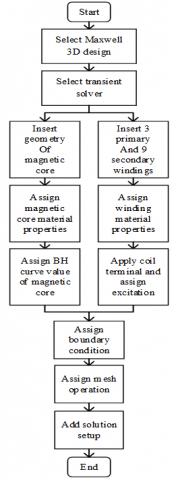Figure 1. Flow chart of 3D modeling of ST

After applying boundary condition and excitation, solution setup has been specified. The problem has been solved according to specified data. Hence, to simulate problem and investigate core loss, transient solver has been selected and for accurate result assign mesh in all elements of geometry of ST. More numbers of mesh will give more precise results. Figure 2 shows solution procedure to find out core loss in Maxwell. ANSYS Maxwell will plot characteristics, from that it will take mean value and display core loss as well as flux distribution.

## 2.png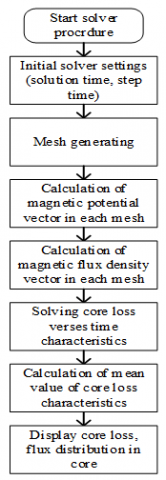Figure 2. Flow chart of solution procedure in Maxwell

5. Result Analysis

Result analysis of the two different configurations of Sen Transformer is presented here. One is a simple structure and another is based on Magnetic Equivalent Circuit (MEC). Both structures are modeled considering their application in distribution side. The flux distribution is not uniform through out in magnetic core due to non linear characteristic of core material. It can be observed that the flux density is larger near core and decreases as we move away from core. This is clearly seen in the results. A 3D modeling of simple ST, Mesh operation, flux distribution of simple ST with sinusoidal input, flux distribution of simple ST with non-sinusoidal input are represented as per Figures 3, 4, 5 and 6, respectively.

5.1 Geometry of simple ST

## 3.png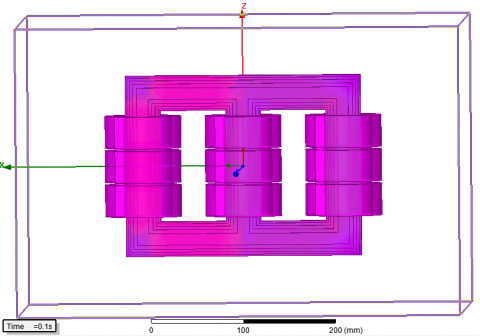Figure 3. 3D modeling of simple ST

## 4.png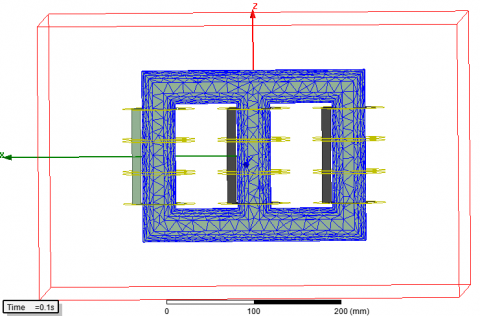Figure 4. Mesh operation of simple ST

## 5.png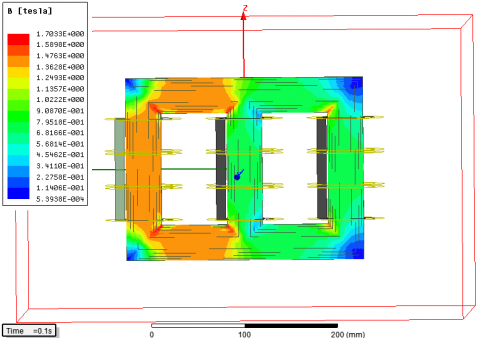Figure 5. Flux distribution of simple ST with sinusoidal input

## 6.png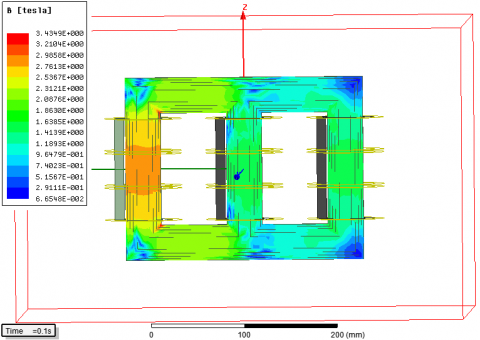Figure 6. Flux distribution of simple ST with non-sinusoidal input

5.2 Geometry of MEC based ST

## 7.pngFigure 7. 3D modeling of MEC based ST

## 8.png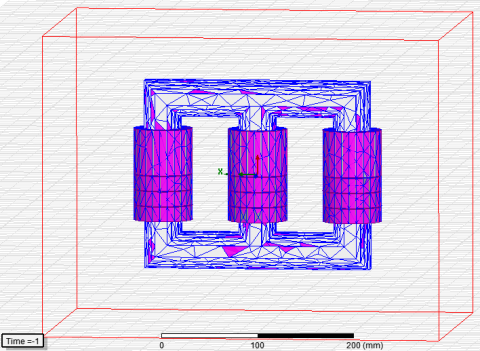Figure 8. Mesh operation of MEC based ST

## 9.pngFigure 9. Flux distribution of MEC based ST with sinusoidal input

## 10.png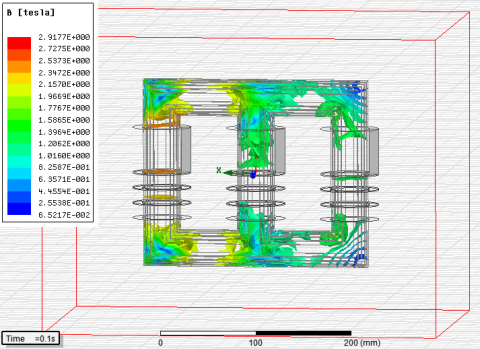Figure 10. Flux distribution of MEC based ST with non-sinusoidal input

A 3D modeling of MEC based ST, Mesh operation, flux distribution of MEC based ST with sinusoidal input, flux distribution of MEC based ST with non-sinusoidal input are represented as per Figures 7, 8, 9 and 10, respectively.

Table 7. Variation of flux density under different input condition

 Simple ST MEC based ST Voltage profile Maximum flux density (Tesla) Average flux density (Tesla) Maximum flux density (Tesla) Average flux density (Tesla) Sinusoidal input 1.7 1.5 1.65 1.45 Non sinusoidal input 3.43 2.37 2.91 1.95

Table 8. No load loss of ST under different input condition

 No load loss (watt) Pnonsin - PSin x 100 Psin Voltage profile Simple ST MEC based ST Simple ST MEC based ST Sinusoidal input 79 75 - - Non sinusoidal input 127 112 60.75% 49.33%

It is observed from Table 7, maximum flux density is 1.65 T in MEC based ST under sinusoidal input and 1.7 T in  simple ST. It is also comparable that very high flux density in non sinusoidal input in both the geometry which is 2.91 and 3.43 for MEC based ST and simple ST respectively. No load loss has been calculated under different input condition i.e. sinusoidal as well as non sinusoidal which is tabulated in Table 8. For sinusiodal input, no load loss in MEC based ST is 75W and in simple ST it is 78W and for non sinusiodal input, no load loss in MEC based ST is 112W and in simple ST it is 127W due to that MEC based structure is preferred for application of ST in distribution system.

5.3 Experimental set up and its result

It is observed that MEC based ST shows less no load loss as compared to simple ST in simulation results. Based on that 0.6 kVA rated MEC based ST have been designed and verified the simulation results. Hardware setup is shown in Figure 11, and experimental result is shown in tabulated form (Table 9) under sinusoidal as well as non sinusoidal case, that gives a result of no load loss in percentage ratio of sinusoidal to non sinusoidal input. In MEC based ST, 18W and 27.25W no load loss occurs while applying sinusoidal and non sinusodal input voltage. Non sinusodal voltage is applied by inserting harmonics in the supply voltage.

## 11.jpg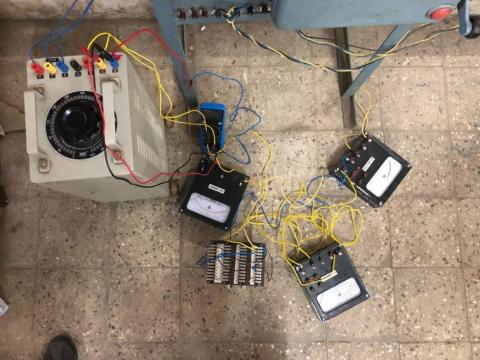Figure 11. Hardware setup of MEC based ST

Table 9. Hardware result of No load loss of MEC based ST under different input condition

 Voltage profile No load loss (watt) MEC based ST (No load loss) Pnonsin - PSinx 100 Psin Sinusoidal case 18 - Non sinusoidal case 27.25 51.38%
6. Conclusion

The Sen Transformer is used as power flow controller and it is connected in the electrical network for 24 hours. As the primary winding of ST is continuously energized from the line, the no-load loss permanently occurs in it. Due to that loss of energy continuously occurs in ST. The core loss has been investigated not only with sinusoidal input supply but also with non sinusoidal input supply to check performance of ST in all conditions. According to ANSYS simulation results, it is observed that with variations in input voltage supply, core loss is varying and distribution of flux density in core becomes more and more non uniform due to non linear behaviour of core material. This phenomenon causes many undesirable issues in core like excessive temperature rise, partial saturation, short-circuit between laminations, uncertain variation of hot spot in the core. In proposed work, finite element analysis has been carried out on ST to check its performance in all situation. By simulating sinusidal and non sinusoidal voltage effects on ST, no-load loss of ST has been calculated with the use of Ansys Maxwell software. Here two different structure of ST has been simulated for same power rating and it is observed that MEC based ST will perform better and have less no load loss than simple ST, as per simulation as well as hardware results. Therefore, MEC based ST design will give efficient power flow control with minimum losses.

Nomenclature
 Pv Core loss, W Ph Hysteresis core loss, W Pc Eddy-current core loss, W Pe Excess core loss, W Cdc DC component Bm Maximum amplitude of the AC flux component, T f Frequency, Hz Kh Hysteresis core loss coefficient Kc Eddy-current core loss coefficient Ke Excess core loss coefficient Bdc DC flux component Kdc Coefficient considering DC flux bias effects d one lamination sheet thickness Pvi ith point of the core loss on the measured loss characteristics curve, W Bmi ith point of the ac flux on the measured loss characteristics curve, T f0 Testing frequency for loss curve, Hz Pnonsin Power loss during non sinusoidal input, W Psin Power loss during sinusoidal input, W B Magnetic flux density, T H Magnetic field, A/m E Electrical field, V/m D Electrical displacement J Electrical current density, A/m2 Greek symbols $\rho$ electrical charge density v velocity of the medium $\sigma$ electrical conductivity $\mu$ megnetic permeability $\epsilon$ Electric permeability
References

 Sen, K.K., Sen, M.L. (2003). Introducing the family of "Sen" transformers: A set of power flow controlling transformers. IEEE Transactions on Power Delivery, 18(1): 149-157. https://doi.org/10.1109/TPWRD.2002.803725

 Liu, J., Dinavahi, V. (2016). Detailed magnetic equivalent circuit based real-time nonlinear power transformer model on FPGA for electromagnetic transient studies. IEEE Transactions on Industrial Electronics, 63(2): 1191-1202. https://doi.org/10.1109/TIE.2015.2477487

 Enokizono, M., Soda, N. (1997). Finite element analysis of transformer model core with measured reluctivity tensor. IEEE Transactions on Magnetics, 33(5): 4110-4112. https://doi.org/10.1109/20.619679

 Ostrenko, M., Andriienko, B. (2017). Transformer impulse surges calculation by FEM coupled to circuit. IEEE Transactions on Magnetics, 53(6): 1-4. https://doi.org/10.1109/TMAG.2017.2661402

 Fonseca, W.S., Lima, D.S., Nunes, M.V.A., Soeiro, N.S., Lima, A.K.F. (2016). Analysis of electromagnetic stresses and structural integrity on the winding of a transformer under inrush currents conditions. 2016 12th IEEE International Conference on Industry Applications (INDUSCON), Curitiba, Brazil, pp. 1-8. https://doi.org/10.1109/INDUSCON.2016.7874578

 Oliveira, J.C., Tavares, C.E., Apolonio, R., Vasconcellos, A.B., Bronzeado, H.S. (2006). Transformer controlled switching to eliminate inrush current - part I: Theory and laboratory validation. 2006 IEEE/PES Transmission & Distribution Conference and Exposition: Latin America, Caracas, Venezuela, pp. 1-5. https://doi.org/10.1109/TDCLA.2006.311523

 Fuchs, E.F., Lin, D.S., Martynaitis, J. (2006). Measurement of three-phase transformer derating and reactive power demand under nonlinear loading conditions. IEEE Transactions on Power Delivery, 21(2): 665-672. https://doi.org/10.1109/TPWRD.2005.858744

 Yu, C.H., Basak, A. (1993). Optimum design of transformer cores by analysing flux and iron loss with the aid of a novel software. IEEE Transactions on Magnetics, 29(2): 1446-1449. https://doi.org/10.1109/20.250675

 Stadler, A., Albach, M. (2006). The influence of the winding layout on the core losses and the leakage inductance in high frequency transformers. IEEE Transactions on Magnetics, 42(4): 735-738. https://doi.org/10.1109/TMAG.2006.871383

 Lin, D.S., Fuchs, E.F. (2006). Real-time monitoring of iron-core and copper losses of transformers Under (Non) Sinusoidal operation. IEEE Transactions on Power Delivery, 21(3): 1333-1341. https://doi.org/10.1109/TPWRD.2006.874118

 Hernández, C., Arjona, M.A. (2007). Design of distribution transformers based on a knowledge-based system and 2D finite elements. Finite Elements in Analysis and Design, 43(8): 659-665. https://doi.org/10.1016/j.finel.2007.01.004

 Yazdani-Asrami, M., Mirzaie, M., Akmal, A.A.S. (2013). No-load loss calculation of distribution transformers supplied by nonsinusoidal voltage using three-dimensional finite element analysis. Energy, 50: 205-219. https://doi.org/10.1016/j.energy.2012.09.050